# Hypernetworks

A hypernetwork is a neural network that produces the weights of another network. As such, it can be seen as a specific type of main network (aka neural network). Therefore, each hypernetwork has a specific interface hypnettorch.hnets.hnet_interface.HyperNetInterface which is derived from the main network interface hypnettorch.mnets.mnet_interface.MainNetInterface.

Note

All hypernetworks in this subpackage implement the abstract interface hypnettorch.hnets.hnet_interface.HyperNetInterface to provide a consistent interface for users.

## Hypernetwork Interface

The module hypnettorch.hnets.hnet_interface contains an interface for hypernetworks.

A hypernetworks is a special type of neural network that produces the weights of another neural network (called the main or target networks, see hypnettorch.mnets.mnet_interface). The name “hypernetworks” was introduced in

Ha et al., “Hypernetworks”, 2016. <https://arxiv.org/abs/1609.09106>

The interface ensures that we can consistently use different types of these networks without knowing their specific implementation details (as long as we only use functionalities defined in class HyperNetInterface).

class hypnettorch.hnets.hnet_interface.HyperNetInterface[source]

A general interface for hypernetworks.

This method simply adds a perturbation dparams (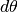) to the unconditional parameters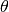.

Parameters:
• dparams (list) – List of tensors.

• params (list, optional) – List of tensors. If unspecified, attribute unconditional_params is taken instead. Otherwise, the method simply returns params + dparams.

Returns:

List were elements of dparams and unconditional params (or params) are summed together.

Return type:

(list)

property conditional_param_shapes

A list of lists of integers denoting the shape of every parameter tensor belonging to the conditional parameters associated with this hypernetwork (i.e., the complement of those returned by unconditional_param_shapes). Note, the returned list is a subset of the shapes maintained in hypnettorch.mnets.mnet_interface.MainNetInterface.param_shapes and is independent whether these parameters are internally maintained (i.e., occuring within conditional_params).

Type:

list

property conditional_param_shapes_ref

A list of integers that has the same length as conditional_param_shapes. Each entry represents an index within attribute hypnettorch.mnets.mnet_interface.MainNetInterface.param_shapes.

It can be used to gain access to meta information about conditional parameters via attribute hypnettorch.mnets.mnet_interface.MainNetInterface.param_shapes_meta.

Type:

list

property conditional_params

The complement of the internally maintained parameters hold by attribute unconditional_params.

A typical example of these parameters are embedding vectors. In continual learning, for instance, there could be a separate task- embedding per task used as hypernet input, see

von Oswald et al., “Continual learning with hypernetworks”, ICLR 2020. https://arxiv.org/abs/1906.00695

Note

This attribute is None if there are no conditional parameters that are internally maintained.

Type:

list or None

convert_out_format(hnet_out, src_format, trgt_format)[source]

Convert the hypernetwork output into another format.

This is a helper method to easily convert the output of a hypernetwork into different formats. Cf. argument ret_format of method forward().

Parameters:
Returns:

The input hnet_out converted into the

target format trgt_format.

Return type:

(list or torch.Tensor)

abstract forward(uncond_input=None, cond_input=None, cond_id=None, weights=None, distilled_params=None, condition=None, ret_format='squeezed')[source]

Perform a pass through the hypernetwork.

Parameters:
• uncond_input (optional) –

The unconditional input to the hypernetwork.

Note

Not all scenarios require a hypernetwork with unconditional inputs. For instance, a task-conditioned hypernetwork only receives a task-embedding (a conditional input) as input.

• cond_input (optional) – If applicable, the conditional input to the hypernetwork.

• cond_id (int or list, optional) –

The ID of the condition to be applied. Only applicable if conditional inputs/weights are maintained internally and conditions are discrete.

Can also be a list of IDs if a batch of weights should be produced.

Condition IDs have to be between 0 and num_conditions.

Note

Option is mutually exclusive with option cond_input.

• weights (list or dict, optional) –

List of weight tensors, that are used as hypernetwork parameters. If not all weights are internally maintained, then this argument is non-optional.

If a list is provided, then it either has to match the length of hypnettorch.mnets.mnet_interface.MainNetInterface.hyper_shapes_learned (if specified) or the length of attribute hypnettorch.mnets.mnet_interface.MainNetInterface.param_shapes.

If a dict is provided, it must have at least one of the following keys specified: - 'uncond_weights' (list): Contains unconditional weights. - 'cond_weights' (list): Contains conditional weights.

• distilled_params (optional) – See docstring of method hypnettorch.mnets.mnet_interface.MainNetInterface.forward().

• condition (optional) – See docstring of method hypnettorch.mnets.mnet_interface.MainNetInterface.forward().

• ret_format (str) –

The format in which the generated weights are returned. The following options are available.

• 'flattened': The hypernet output will be a tensor of shape [batch_size, num_outputs] (see num_outputs).

• 'sequential': A list of length batch size is returned that contains lists of length len(target_shapes), which contain tensors with shapes determined by attribute target_shapes. Hence, each entry of the returned list contains the weights for one sample in the input batch.

• 'squeezed': Same as 'sequential', but if the batch size is 1, the list will be unpacked, such that a list of tensors is returned (rather than a list of list of tensors).

Example

Assume target_shapes to be [[10, 5], ] and cond_input to be the only input to the hypernetwork, which is a batch of embeddings [B, E], where B is the batch size and E is the embedding size.

Note, num_outputs = 60 in this case (cmp. num_outputs).

If 'flattened' is used, a tensor of shape [B, 60] is returned. If 'sequential' or 'squeezed' is used and B > 1 (e.g., B=3), then a list of lists of tensors (here denoted by their shapes) is returned [[[10, 5], ], [[10, 5], ], [[10, 5], ]]. However, if B == 1 and 'squeezed' is used, then a list of tensors is returned, e.g., [[10, 5], ].

Returns:

See description of argument ret_format.

Return type:

(list or torch.Tensor)

Returns the task_id-th element from attribute conditional_params.

Deprecated since version 1.0: Please access elements of attribute conditional_params directly, as the conditional parameters do not have to correspond to task embeddings.

Parameters:

task_id (int) – Determines which element of conditional_params should be returned.

Returns:

(torch.nn.Parameter)

Returns attribute conditional_params.

Deprecated since version 1.0: Please access attribute conditional_params directly, as the conditional parameters do not have to correspond to task embeddings.

Returns:

(list or None)

property num_known_conds

The number of conditions known to this hypernetwork. If the number of conditions is discrete and internally maintained by the hypernetwork, then this attribute specifies how many conditions the hypernet manages.

Note

The option does not have to agree with the length of attribute conditional_params. For instance, in certain cases there are multiple conditional weights maintained per condition.

Type:

int

property num_outputs

The total number of output neurons (number of weights generated for the target network). This quantity can be computed based on attribute target_shapes.

Type:

int

property target_shapes

A list of list of integers representing the shapes of weight tensors generated, i.e., the hypernet output, which could be, for instance, the mnets.mnet_interface.MainNetInterface.hyper_shapes_learned of another network whose weights this hypernetwork is producing.

Type:

list

property unconditional_param_shapes

A list of lists of integers denoting the shape of every parameter tensor belonging to the unconditional parameters associated with this hypernetwork. Note, the returned list is a subset of the shapes maintained in hypnettorch.mnets.mnet_interface.MainNetInterface.param_shapes and is independent whether these parameters are internally maintained (i.e., occuring within unconditional_params).

Type:

list

property unconditional_param_shapes_ref

A list of integers that has the same length as unconditional_param_shapes. Each entry represents an index within attribute hypnettorch.mnets.mnet_interface.MainNetInterface.param_shapes.

Type:

list

property unconditional_params

Internally maintained parameters of the hypernetwork excluding parameters that may be specific to a given condition, e.g., task embeddings in continual learning.

Hence, it is the portion of parameter tensors from attribute mnets.mnet_interface.MainNetInterface.internal_params that is not specific to a certain task/condition.

Note

This attribute is None if there are no unconditional parameters that are internally maintained.

Example

An example use-case for a hypernetwork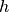could be the following: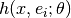, where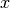is an arbitrary input,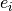is a learned embedding (condition) andare the internal “unconditional” parameters of the hypernetwork. In some cases (for simplicity), the conditionsas well as the parametersare maintained internally by this class. This attribute can be used to gain access to the “unconditional” parameters, while mnets.mnet_interface.MainNetInterface.internal_params would return all “conditional” parametersas well as the “unconditional” parameters.

Type:

list or None

property unconditional_params_ref

A list of integers that has the same length as unconditional_params. Each entry represents an index within attribute hypnettorch.mnets.mnet_interface.MainNetInterface.internal_params.

If unconditional_params is None, the this attribute is None as well.

Example

Using an instance hnet that implements this interface, the following is True.

hnet.internal_params[hnet.unconditional_params_ref[i]] is                 hnet.unconditional_params[i]


Note

This attribute has different semantics compared to unconditional_param_shapes_ref which points to locations within hypnettorch.mnets.mnet_interface.MainNetInterface.param_shapes, wheras this attribute points to locations within hypnettorch.mnets.mnet_interface.MainNetInterface.internal_params.

Type:

list or None

## Chunked Deconvolutional Hypernetwork with Self-Attention Layers

The module hnets.chunked_deconv_hnet implements a chunked version of the transpose convolutional hypernetwork represented by class hnets.deconv_hnet.HDeconv (similar as to hnets.chunked_mlp_hnet.ChunkedHMLP represents a chunked version of the full hypernetwork hnets.mlp_hnet.HMLP).

Therefore, an instance of class ChunkedHDeconv manages internally an instance of class hnets.deconv_hnet.HDeconv, which is invoked multiple time with a different additional input (the so called chunk embedding) to produce a chunk of the target weights at a time, which are later put together. See description of module hnets.chunked_mlp_hnet for more details.

Note

This type of hypernetwork is completely agnostic to the architecture of the target network. The splits happen at arbitrary locations in the flattened target network weight vector.

class hypnettorch.hnets.chunked_deconv_hnet.ChunkedHDeconv(target_shapes, hyper_img_shape, chunk_emb_size=8, cond_chunk_embs=False, uncond_in_size=0, cond_in_size=8, num_layers=5, num_filters=None, kernel_size=5, sa_units=(1, 3), verbose=True, activation_fn=ReLU(), use_bias=True, no_uncond_weights=False, no_cond_weights=False, num_cond_embs=1, use_spectral_norm=False, use_batch_norm=False)[source]

Implementation of a chunked deconvolutional hypernet.

The target_shapes will be flattened and split into chunks of size chunk_size = np.prod(hyper_img_shape). In total, there will be np.ceil(self.num_outputs/chunk_size) chunks, where the last chunk produced might contain a remainder that is discarded.

Each chunk has it’s own chunk embedding that is fed into the underlying hypernetwork.

Note

It is possible to set uncond_in_size and cond_in_size to zero if cond_chunk_embs is True.

(....)

See attributes of class hnets.chunked_mlp_hnet.ChunkedHMLP.

Parameters:
• (....) – See constructor arguments of class hnets.deconv_hnet.HDeconv.

• chunk_emb_size (int) – See constructor arguments of class hnets.chunked_mlp_hnet.ChunkedHMLP.

• cond_chunk_embs (bool) – See constructor arguments of class hnets.chunked_mlp_hnet.ChunkedHMLP.

Initializes internal Module state, shared by both nn.Module and ScriptModule.

property chunk_emb_size

Getter for read-only attribute chunk_emb_size.

property cond_chunk_embs

Getter for read-only attribute cond_chunk_embs.

forward(uncond_input=None, cond_input=None, cond_id=None, weights=None, distilled_params=None, condition=None, ret_format='squeezed')[source]

Compute the weights of a target network.

Parameters:

(....) – See docstring of method hnets.chunked_mlp_hnet.ChunkedHMLP.forward().

Returns:

See docstring of method hnets.hnet_interface.HyperNetInterface.forward().

Return type:

(list or torch.Tensor)

get_chunk_emb(chunk_id=None, cond_id=None)[source]

Get the chunk_id-th chunk embedding.

Parameters:

(....) – See docstring of method hnets.chunked_mlp_hnet.ChunkedHMLP.get_chunk_emb().

Returns:

(torch.nn.Parameter)

get_cond_in_emb(cond_id)[source]

Get the cond_id-th (conditional) input embedding.

Parameters:

(....) – See docstring of method hnets.deconv_hnet.HDeconv.get_cond_in_emb().

Returns:

(torch.nn.Parameter)

property num_chunks

Getter for read-only attribute num_chunks.

training: bool

## Chunked MLP - Hypernetwork

The module hnets.chunked_mlp_hnet contains a Chunked Hypernetwork, that uses a full hypernetwork (see hnets.mlp_hnet.HMLP) to produce one chunk of the output weights at a time.

The hypernetwork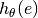(with input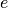) operates as follows. The target outputs (see hnets.hnet_interface.HyperNetInterface.target_shapes) are flattened and split into equally sized chunks. Those chunks are separately generated by an internal full hypernetwork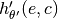(that is hidden from the user), where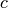denotes the chunk embedding, which are internally maintained and chunk-specific.

Note

This type of hypernetwork is completely agnostic to the architecture of the target network. The splits happen at arbitrary locations in the flattened target network weight vector.

class hypnettorch.hnets.chunked_mlp_hnet.ChunkedHMLP(target_shapes, chunk_size, chunk_emb_size=8, cond_chunk_embs=False, uncond_in_size=0, cond_in_size=8, layers=(100, 100), verbose=True, activation_fn=ReLU(), use_bias=True, no_uncond_weights=False, no_cond_weights=False, num_cond_embs=1, dropout_rate=-1, use_spectral_norm=False, use_batch_norm=False)[source]

Implementation of a chunked fully-connected hypernet.

The target_shapes will be flattened and split into chunks of size chunk_size. In total, there will be np.ceil(self.num_outputs/chunk_size) chunks, where the last chunk produced might contain a remainder that is discarded.

Each chunk has it’s own chunk embedding that is fed into the underlying hypernetwork.

Note

It is possible to set uncond_in_size and cond_in_size to zero if cond_chunk_embs is True.

Parameters:
• (....) – See constructor arguments of class hnets.mlp_hnet.HMLP.

• chunk_size (int) – The chunk size, i.e, the number of weights produced by individual forward passes of the internally maintained instance of a full hypernet (see hnets.mlp_hnet.HMLP) upon receiving a chunk embedding).

• chunk_emb_size (int) – The size of a chunk embedding.

• cond_chunk_embs (bool) –

Whether chunk embeddings are unconditional (False) or conditional (True) parameters. See constructor argument cond_chunk_embs.

Note

Embeddings will be initialized with a normal distribution using zero mean and unit variance.

• cond_chunk_embs

Consider chunk embeddings to be conditional. In this case, there will be a different set of chunk embeddings per condition (specified via num_cond_embs).

If False, there will be a total of num_chunks chunk embeddings that are maintained within hnets.hnet_interface.HyperNetInterface.unconditional_param_shapes. If True, there will be num_cond_embs * self.num_chunks chunk embeddings that are maintained within hnets.hnet_interface.HyperNetInterface.conditional_param_shapes. However, if num_cond_embs == 0, then chunk embeddings have to be provided in a special way to the forward() method (see the corresponding argument weights).

Initializes internal Module state, shared by both nn.Module and ScriptModule.

apply_chunked_hyperfan_init(method='in', use_xavier=False, uncond_var=1.0, cond_var=1.0, eps=1e-05, cemb_normal_init=False, mnet=None, target_vars=None)[source]

Initialize the network using a chunked hyperfan init.

Inspired by the method Hyperfan Init which we implemented for the MLP hypernetwork in method hnets.mlp_hnet.HMLP.apply_hyperfan_init(), we heuristically developed a better initialization method for chunked hypernetworks.

Unfortunately, the Hyperfan Init method from the paper does not apply to this kind of hypernetwork, since we reuse the same hypernet output head for the whole main network.

Luckily, we can provide a simple heuristic. Similar to Meyerson & Miikkulainen we play with the variance of the input embeddings to affect the variance of the output weights.

In a chunked hypernetwork, the input for each chunk is identical except for the chunk embeddings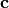. Letdenote the remaining inputs to the hypernetwork, which are identical for all chunks. Then, assuming the hypernetwork was initialized via fan-in init, the variance of the hypernetwork output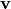can be written as follows (see documentation of method hnets.mlp_hnet.HMLP.apply_hyperfan_init()):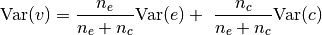Hence, we can achieve a desired output variance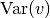by initializing the chunk embeddingsvia the following variance: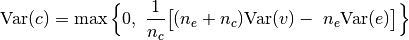Now, one important question remains. How do we pick a desired output variancefor a chunk?

Note, a chunk may include weights from several layers. The likelihood for this to happen depends on the main net architecture and the chunk size (see constructor argument chunk_size). The smaller the chunk size, the less likely it is that a chunk will contain elements from multiple main net weight tensors.

In case each chunk would contain only weights from one main net weight tensor, we could simply pick the variancethat would have been chosen by a main net initialization method (such as Xavier).

In case a chunk contains contributions from several main net weight tensors, we apply the following heuristic. If a chunk contains contributions of a set of main network weight tensors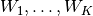with relative contribution sizes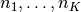such thatwhere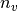denotes the chunk size and if the corresponding main network initialization method would require init variances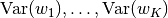, then we simply request a weighted average as follow: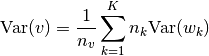What about bias vectors? Usually, the variance analysis applied to Xavier or Kaiming init assumes that biases are initialized to zero. This is not possible in this setting, as it would require assigning a negative variance to. Instead, we follow the default PyTorch initialization (e.g., see method reset_parameters in class torch.nn.Linear). There, bias vectors are initialized uniformly within a range of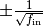where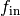refers to the fan-in of the layer. This type of initialization corresponds to a variance of.

Note

All hypernet inputs are assumed to be zero-mean random variables.

Note

To avoid that the variances with which chunks are initialized have to be clipped (because they are too small or even negative), the variance of the remaining hypernet inputs should be properly scaled. In general, one should adhere the following rule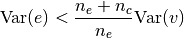This method will calculate and print the maximum value that should be chosen for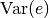and will print warnings if variances have to be clipped.

Parameters:
• (....) – See arguments of method hnets.mlp_hnet.HMLP.apply_hyperfan_init().

• method (str) –

The type of initialization that should be applied. Possible options are:

• in: Use Chunked Hyperfan-in, i.e., rather the output variances of the hypernetwork should correspond to fan-in variances.

• out: Use Chunked Hyperfan-out, i.e., rather the output variances of the hypernetwork should correspond to fan-out variances.

• harmonic: Use the harmonic mean of the fan-in and fan-out variance as target variance of the hypernetwork output.

• eps (float) – The minimum variance with which a chunk embedding is initialized.

• cemb_normal_init (bool) – Use normal init for chunk embeddings rather than uniform init.

• target_vars (list or dict, optional) –

The variance of the distribution for each parameter tensor generated by this hypernetwork. Target variance values can either be provided as list of length len(hnet.target_shapes) or as dictionary. The usage is analoguous to the usage of parameter w_val of method hnets.mlp_hnet.HMLP.apply_hyperfan_init().

Note

This method currently does not allow initial output distributions with non-zero mean. However, the docstring of method probabilistic.gauss_hnet_init.gauss_hyperfan_init() describes how this is in principle feasible and might be incorporated in the future.

Note

Unspecified target variances for parameter tensors of type 'weight' or 'bias' are computed as described above. Default target variances for all other parameter tensor types are simply 1.

property chunk_emb_size

See constructor argument chunk_emb_size.

property cond_chunk_embs

See constructor argument cond_chunk_embs.

distillation_targets()[source]

Targets to be distilled after training.

See docstring of abstract super method mnets.mnet_interface.MainNetInterface.distillation_targets().

Returns:

See hnets.mlp_hnet.HMLP.distillation_targets().

forward(uncond_input=None, cond_input=None, cond_id=None, weights=None, distilled_params=None, condition=None, ret_format='squeezed')[source]

Compute the weights of a target network.

Parameters:
• (....) – See docstring of method hnets.mlp_hnet.HMLP.forward().

• weights (list or dict, optional) –

If provided as dict and chunk embeddings are considered conditional (see constructor argument cond_chunk_embs), then the additional key chunk_embs can be used to pass a batch of chunk embeddings. This option is mutually exclusive with the option of passing cond_id. Note, if conditional inputs via cond_input are expected, then the batch sizes must agree.

A batch of chunk embeddings is expected to be tensor of shape [B, num_chunks, chunk_emb_size], where B denotes the batch size.

Returns:

See docstring of method hnets.hnet_interface.HyperNetInterface.forward().

Return type:

(list or torch.Tensor)

get_chunk_emb(chunk_id=None, cond_id=None)[source]

Get the chunk_id-th chunk embedding.

Parameters:
• chunk_id (int, optional) – A number between 0 and num_chunks - 1. If not specified, a full chunk matrix with shape [num_chunks, chunk_emb_size] is returned. Otherwise, the chunk_id-th row is returned.

• cond_id (int) – Is mandatory if constructor argument cond_chunk_embs was set. Determines the set of chunk embeddings to be considered.

Returns:

(torch.nn.Parameter)

get_cond_in_emb(cond_id)[source]

Get the cond_id-th (conditional) input embedding.

Parameters:

(....) – See docstring of method hnets.mlp_hnet.HMLP.get_cond_in_emb().

Returns:

(torch.nn.Parameter)

property num_chunks

The number of chunks that make up the final hypernet output.

This also corresponds to the number of chunk embeddings required per forward sweep.

Type:

int

training: bool

## Deconvolutional Hypernetwork with Self-Attention Layers

The module hnets.deconv_hnet implements a hypernetwork that uses transpose convolutions (like the generator of a GAN) to generate weights. Though, as convolutions usually suffer from only capturing local correlations sufficiently, we incorporate the self-attention mechanism developed by

See utils.self_attention_layer.SelfAttnLayerV2 for details on this layer type.

The purpose of this network can be seen as the convolutional analogue of the fully-connected hnets.mlp_hnet.HMLP. Hence, it produces all weights in one go; and does not utilize chunking to obtain better weight compression ratios (a chunked version can be found in module hnets.chunked_deconv_hnet).

class hypnettorch.hnets.deconv_hnet.HDeconv(target_shapes, hyper_img_shape, uncond_in_size=0, cond_in_size=8, num_layers=5, num_filters=None, kernel_size=5, sa_units=(1, 3), verbose=True, activation_fn=ReLU(), use_bias=True, no_uncond_weights=False, no_cond_weights=False, num_cond_embs=1, use_spectral_norm=False, use_batch_norm=False)[source]

Implementation of a deconvolutional full hypernet.

This is a convolutional network, employing transpose convolutions. The network structure is inspired by the DCGAN generator structure, though, we are additionally using self-attention layers to model global dependencies.

In general, each transpose convolutional layer will roughly double its input size. Though, we set the hard constraint that if the input size of a transpose convolutional layer would be smaller 4, then it doesn’t change the size.

The network allows to maintain a set of embeddings internally that can be used as conditional input (cmp. hnets.mlp_hnet.HMLP).

Parameters:
• (....) – See constructor arguments of class hnets.mlp_hnet.HMLP.

• hyper_img_shape (tuple) –

Since the network has a (de-)convolutional output layer, the output will be in an image-like shape. Therefore, it won’t be possible to precisely produce the number of weights prescribed by target_shapes. Therefore, the hyper-image size defined via this option has to be chosen big enough, i.e., the number of pixels must be greater equal than the number of weights to be produced. Remaining pixels will be discarded.

This option has to be a tuple (width, height), denoting the internal output shape of the the hypernet. The number of output channels is assumed to be 1, except if specified otherwise via (width, height, channels).

• num_layers (int) – The number of transpose convolutional layers including the initial fully-connected layer.

• num_filters (list, optional) –

List of integers of length num_layers-1. The number of output channels in each hidden transpose conv. layer. By default, the number of filters in the last hidden layer will be 128 and doubled in every prior layer.

Note

The output of the first layer (which is fully-connected) is here considered to be in the shape of an image tensor.

• kernel_size (int, tuple or list, optional) – A single number, a tuple (k_x, k_y) or a list of scalars/tuples of length num_layers-1. Specifying the kernel size in each convolutional layer.

• sa_units (tuple or list) – List of integers, each representing the index of a layer in this network after which a self-attention unit should be inserted. For instance, index 0 represents the fully-connected layer. The last layer may not be chosen.

Initializes internal Module state, shared by both nn.Module and ScriptModule.

distillation_targets()[source]

Targets to be distilled after training.

See docstring of abstract super method mnets.mnet_interface.MainNetInterface.distillation_targets().

This network does not have any distillation targets.

Returns:

None

forward(uncond_input=None, cond_input=None, cond_id=None, weights=None, distilled_params=None, condition=None, ret_format='squeezed')[source]

Compute the weights of a target network.

Parameters:

(....) – See docstring of method hnets.mlp_hnet.HMLP.forward().

Returns:

See docstring of method hnets.hnet_interface.HyperNetInterface.forward().

Return type:

(list or torch.Tensor)

get_cond_in_emb(cond_id)[source]

Get the cond_id-th (conditional) input embedding.

Parameters:

cond_id (int) – Determines which input embedding should be returned (the ID has to be between 0 and num_cond_embs-1, where num_cond_embs denotes the corresponding constructor argument).

Returns:

(torch.nn.Parameter)

training: bool

## Hypernetwork-container that wraps a mixture of hypernets

The module hnets.hnet_container contains a hypernetwork container, i.e., a hypernetwork that produces weights by internally using a mixture of hypernetworks that implement the interface hnets.hnet_interface.HyperNetInterface. The container also allows the specification of shared or condition-specific weights.

Example

Assume a target network with shapes target_shapes=[[10, 5], , , , [5, 5]], where the first 4 tensors represent the weight matrix, bias vector and batch norm scale and shift, while the last tensor is the linear output layer’s weight matrix.

We consider two usecase scenarios. In the first one, the first layer weights (matrix and bias vector) are generated by a hypernetwork, while the batch norm weights should be realized via a fixed set of shared weights. The output weights shall be condition-specific:

from hnets import HMLP

# First-layer weights.
fl_hnet = HMLP([[10, 5], ], num_cond_embs=5)

def assembly_fct(list_of_hnet_tensors, uncond_tensors, cond_tensors):
assert len(list_of_hnet_tensors) == 1
return list_of_hnet_tensors + uncond_tensors + cond_tensors

hnet = HContainer([[10, 5], , , , [5, 5]], assembly_fct,
hnets=[fl_hnet], uncond_param_shapes=[, ],
cond_param_shapes=[[5, 5]],
uncond_param_names=['bn_scale', 'bn_shift'],
cond_param_names=['weight'], num_cond_embs=5)


In the second usecase scenario, we utilize two separate hypernetworks, one as above and a second one for the condition-specific output weights. Batchnorm weights remain to be realized via a single set of shared weights.

from hnets import HMLP

# First-layer weights.
fl_hnet = HMLP([[10, 5], ], num_cond_embs=5)
# Last-layer weights.
ll_hnet = HMLP([[5, 5]], num_cond_embs=5)

def assembly_fct(list_of_hnet_tensors, uncond_tensors, cond_tensors):
assert len(list_of_hnet_tensors) == 2
return list_of_hnet_tensors + uncond_tensors + \
list_of_hnet_tensors

hnet = HContainer([[10, 5], , , , [5, 5]], assembly_fct,
hnets=[fl_hnet, ll_hnet],
uncond_param_shapes=[, ],
uncond_param_names=['bn_scale', 'bn_shift'],
num_cond_embs=5)

class hypnettorch.hnets.hnet_container.HContainer(target_shapes, assembly_fct, hnets=None, uncond_param_shapes=None, cond_param_shapes=None, uncond_param_names=None, cond_param_names=None, verbose=True, no_uncond_weights=False, no_cond_weights=False, num_cond_embs=1)[source]

Implementation of a wrapper that abstracts the use of a set of hypernetworks.

Note

Parameter tensors instantiated by this constructor are initialized via a normal distribution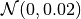.

Parameters:
• (....) – See constructor arguments of class hnets.mlp_hnet.HMLP.

• assembly_fct (func) –

A function handle that takes the produced tensors of each internal hypernet (see arguments hnets, uncond_param_shapes and cond_param_shapes) and converts them into tensors with shapes target_shapes.

The function handle must have the signature: assembly_fct(list_of_hnet_tensors, uncond_tensors, cond_tensors) . The first argument is a list of lists of tensors, the reamining two are lists of tensors. hnet_tensors contains the output of each hypernetwork in hnets. uncond_tensors contains all internally maintained unconditional weights as specified by uncond_param_shapes. cond_tensors contains the internally maintained weights corresponding to the selected condition and as specified by argument cond_param_shapes. The function is expected to return a list of tensors, each of them having a shape as specified by target_shapes.

Example

Assume target_shapes=[, , [10, 5], ] and that hnets is made up of two hypernetworks with output shapes [] and [, [10, 5]]. In addition cond_param_shapes=[]. Then the argument hnet_tensors will be a list of lists of tensors as follows: [[tensor(3)], [tensor(3), tensor(10, 5)], uncond_tensors will be an empty list and cond_tensors will be list of tensors: [[tensor(5)]].

The output of assembly_fct is expected to be a list of tensors as follows: [tensor(3), tensor(3), tensor(10, 5), tensor(5)].

Note

This function considers one sample at a time, even if a batch of inputs is processed.

Note

It is assumed that assembly_fct does not further process the incoming weights. Otherwise, the attributes mnets.mnet_interface.MainNetInterface.has_fc_out and mnets.mnet_interface.MainNetInterface.has_linear_out might be invalid.

• hnets (list, optional) – List of instances of class hnets.hnet_interface.HyperNetInterface. All these hypernetworks are assumed to produce a part of the weights that are then assembled to a common hypernetwork output via the assembly_fct.

• uncond_param_shapes (list, optional) –

List of lists of integers. Each entry in the list encodes the shape of an (unconditional) parameter tensor that will be added to attribute hnets.hnet_interface.HyperNetInterface.unconditional_params and additionally will also become an output of this hypernetwork that is passed to the assembly_fct.

Hence, these parameters are independent of the hypernetwork input. Thus, they are just treated as normal weights as if they were part of the main network. This option therefore only provides the convinience of mimicking the behavior weights would elicit if they were part of the main network without needing to change the main network its implementation.

• cond_param_shapes (list, optional) –

List of lists of integers. Each entry in the list encodes the shape of a (conditional) parameter tensor that will be added to attribute hnets.hnet_interface.HyperNetInterface.conditional_params (how often it will be added is determined by argument num_cond_embs). It is otherwise similar to option uncond_param_shapes.

Note

If this option is specified, then argument cond_id of forward() has to be specified.

• uncond_param_names (list, optional) –

If provided, it must have the same length as uncond_param_shapes. It will contain a list of strings that are used as values for key name in attribute hnets.hnet_interface.HyperNetInterface.param_shapes_meta.

If not provided, shapes with more than 1 element are assigned value weights and all others are assigned value bias.

• cond_param_names (list, optional) – Same as argument uncond_param_names for argument cond_param_shapes.

Initializes internal Module state, shared by both nn.Module and ScriptModule.

distillation_targets()[source]

Targets to be distilled after training.

See docstring of abstract super method mnets.mnet_interface.MainNetInterface.distillation_targets().

This network does not have any distillation targets.

Returns:

None

forward(uncond_input=None, cond_input=None, cond_id=None, weights=None, distilled_params=None, condition=None, ret_format='squeezed')[source]

Compute the weights of a target network.

Parameters:
• (....) – See docstring of method hnets.mlp_hnet.HMLP.forward(). Some further information is provided below.

• uncond_input (optional) – Passed to underlying hypernetworks (see constructor argument hnets).

• cond_input (optional) – Passed to underlying hypernetworks (see constructor argument hnets).

• cond_id (int or list, optional) – Only passed to underlying hypernetworks (see constructor argument hnets) if cond_input is None.

• weights (list or dict, optional) –

If provided as dict then an additional key hnets can be specified, which has to a list of the same length as the constructor argument hnets containing dictionaries as entries that will be concatenated to the extracted (hnet-specific) keys uncond_weights and cond_weights.

For instance, for an instance of class hnets.chunked_mlp_hnet.ChunkedHMLP the additional key chunk_embs might be added.

• condition (optional) – Will be passed to the underlying hypernetworks (see constructor argument hnets).

Returns:

See docstring of method hnets.hnet_interface.HyperNetInterface.forward().

Return type:

(list or torch.Tensor)

property internal_hnets

The list of internal hypernetworks provided via constructor argument hnets.

If hnets was not provided, the attribute is an empty list.

Type:

list

training: bool

## Helper functions for hypernetworks

The module hnets.hnet_helpers contains utilities that should simplify working with hypernetworks that implement the interface hnets.hnet_interface.HyperNetInterface. Those helper functions are meant to handle common manipulations (such as embedding initialization) in an abstract way that hides implementation details to the user.

hypnettorch.hnets.hnet_helpers.get_conditional_parameters(hnet, cond_id)[source]

Get condition specific parameters from the hypernetwork.

Example

Class hnets.mlp_hnet.HMLP may only have one embedding (the conditional input embedding) per condition as conditional parameter. Thus, this function will simply return [hnet.get_cond_in_emb(cond_id)].

Parameters:
• hnet (hnets.hnet_interface.HyperNetInterface) – The hypernetwork whose conditional parameters regarding cond_id should be extraced.

• cond_id (int) – The condition (or its conditional ID) for which parameters should be extraced.

Returns:

A list of tensors, a subset of attribute

hnets.hnet_interface.HyperNetInterface.conditional_params, that are specific to the condition cond_id. An empty list is returned if conditional parameters are not maintained internally.

Return type:

(list)

hypnettorch.hnets.hnet_helpers.init_chunk_embeddings(hnet, normal_mean=0.0, normal_std=1.0, init_fct=None)[source]

Initialize chunk embeddings.

This function only applies to hypernetworks that make use of chunking, such as hnets.chunked_mlp_hnet.ChunkedHMLP. All other hypernetwork types will be unaffected by this function.

This function handles the initialization of embeddings very similar to function init_conditional_embeddings(), except that the function handle init_fct has a slightly different signature. It receives two positional arguments, the chunk embedding and the chunk embedding ID as well as one optional argument cond_id, the conditional ID (in case of conditional chunk embeddings).

init_fct = lambda cemb, cid, cond_id=None : nn.init.constant_(cemb, 0)


Note

Class hnets.structured_mlp_hnet.StructuredHMLP has multiple sets of chunk tensors as specified by attribute hnets.structured_mlp_hnet.StructuredHMLP.chunk_emb_shapes. As a simplifying design choice, the tensors passed to init_fct will not be single embeddings (i.e., vectors), but tensors of embeddings according to the shapes in attribute hnets.structured_mlp_hnet.StructuredHMLP.chunk_emb_shapes.

Parameters:

(....) – See docstring of function init_conditional_embeddings().

hypnettorch.hnets.hnet_helpers.init_conditional_embeddings(hnet, normal_mean=0.0, normal_std=1.0, init_fct=None)[source]

Initialize internally maintained conditional input embeddings.

This function initializes conditional embeddings if the hypernetwork has any and they are internally maintained. For instance, the conditional embeddings of an HMLP instance are those returned by the method hnets.mlp_hnet.HMLP.get_cond_in_emb().

By default, those embedding will follow a normal distribution. However, one may pass a custom init function init_fct that receives the embedding and its corresponding conditional ID as input (as is expected to modify the embedding in-place):

init_fct(cond_emb, cond_id)


Hypernetworks that don’t make use of internally maintained conditional input embeddings will not be affected by this function.

Note

Chunk embeddings may also be conditional parameters, but are not considered conditional input embeddings here. Conditional chunk embeddings can be initialized using function init_chunk_embeddings().

Parameters:
• hnet (hnets.hnet_interface.HyperNetInterface) – The hypernetwork whose conditional embeddings should be initialized.

• normal_mean (float) – The mean of the normal distribution with which embeddings should be initialized.

• normal_std (float) – The std of the normal distribution with which embeddings should be initialized.

• init_fct (func, optional) – A function handle that receives a conditional embedding and its ID as input and initializes the embedding in-place. If provided, arguments normal_mean and normal_std will be ignored.

## Hypernetwork-wrapper for input-preprocessing and output-postprocessing

The module hnets.hnet_perturbation_wrapper implements a wrapper for hypernetworks that implement the interface hnets.hnet_interface.HyperNetInterface. By default, the wrapper is meant for perturbing hypernetwork outputs, such that an implicit distribution (realized via a hypernetwork) with low-dimensional support can be inflated to have support in the full weight space.

However, the wrapper allows in general to pass function handles that preprocess inputs and/or postprocess hypernetwork outputs.

class hypnettorch.hnets.hnet_perturbation_wrapper.HPerturbWrapper(hnet, hnet_uncond_in_size=None, sigma_noise=0.02, input_handler=None, output_handler=None, verbose=True)[source]

Hypernetwork wrapper for output perturbation.

This wrapper is meant as a helper for hypernetworks that represent implicit distributions, i.e., distributions that transform a simple base distributioninto a complex target distributions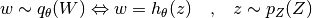However, the wrapper is more versatile and can also become handy in a variety of other use cases. Yet, in the following we concentrate on implicit distributions and their practical challenges. One main challenge is typically that the density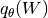is only defined on a lower-dimensional manifold of the weight space. This is often an undesirable property (e.g., such implicit distributions are often not amenable for optimization with standard divergence measures, such as the KL).

A simple way to overcome this issue is to add noise perturbations to the output of the hypernetwork, such that the perturbations itself origin from a full-support distribution. By default, this hypernetwork wrapper adjusts the sampling procedure above in the following way

(1)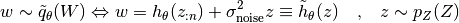where now,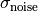is a hyperparameter that controls the perturbation strength, and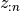are the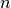first entries of the vector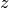.

By default, the unconditional input size of this hypernetwork will be of size hnet.num_outputs (if input_handler is not provided) and the output size will be of the same size.

Parameters:
• hnet (hnets.hnet_interface.HyperNetInterface) – The hypernetwork around which this wrapper should be wrapped.

• hnet_uncond_in_size (int) – This argument refers tofrom Eq. (1). If input_handler is provided, this argument will be ignored.

• sigma_noise (float) – The perturbation strengthfrom Eq. (1). If output_handler is provided, this argument will be ignored.

• input_handler (func, optional) –

A function handler to process the inputs to the hnets.hnet_interface.HyperNetInterface.forward() method of hnet. The function handler should have the following signature

uncond_input_int, cond_input_int, cond_id_int = input_handler( \
uncond_input=None, cond_input=None, cond_id=None)


The returned values will be passed to internal_hnet.

Example

For instance, to reproduce the behavior depicted in Eq. (1) one could provide the following handler

def input_handler(uncond_input=None, cond_input=None,
cond_id=None):
assert uncond_input is not None
n = 5
return uncond_input[:, :n], cond_input, cond_id


• output_handler (func, optional) –

A function handler to postprocess the outputs of the internal hypernetwork internal_hnet.

A function handler with the following signature is expected.

hnet_out = output_handler(hnet_out_int, uncond_input=None,
cond_input=None, cond_id=None)


where hnet_out_int is the output of the internal hypernetwork internal_hnet and the remaining arguments are the original arguments passed to method forward(). hnet_out_int will always have the format ret_format='flattened' and is also expected to return this format.

Example

Deviating from Eq. (1), let’s say we want to implement the following sampling behavior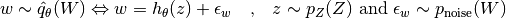In this case the unconditional input uncond_input to the forward() method is expected to have size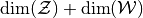.

def input_handler(uncond_input=None, cond_input=None,
cond_id=None):
assert uncond_input is not None
return uncond_input[:, :dim_z], cond_input, cond_id

def output_handler(hnet_out_int, uncond_input=None,
cond_input=None, cond_id=None):
assert uncond_input is not None
return hnet_out_int + uncond_input[:, dim_z:]


• verbose (bool) – Whether network information should be printed during network creation.

Initializes internal Module state, shared by both nn.Module and ScriptModule.

distillation_targets()[source]

Targets to be distilled after training.

See docstring of abstract super method mnets.mnet_interface.MainNetInterface.distillation_targets().

Returns:

Simply returns the distillation_targets of the internal hypernet internal_hnet.

forward(uncond_input=None, cond_input=None, cond_id=None, weights=None, distilled_params=None, condition=None, ret_format='squeezed')[source]

Compute the weights of a target network.

Parameters:

(....) – See docstring of method hnets.hnet_interface.HyperNetInterface.forward().

Returns:

See docstring of method hnets.hnet_interface.HyperNetInterface.forward().

Return type:

(list or torch.Tensor)

property internal_hnet

The underlying hypernetwork that was passed via constructor argument hnet.

Type:

hnets.hnet_interface.HyperNetInterface

training: bool

## MLP - Hypernetwork

The module hnets.mlp_hnet contains a fully-connected hypernetwork (also termed full hypernet).

This type of hypernetwork represents one of the most simplistic architectural choices to realize a weight generator. An embedding input, which may consists of conditional and unconditional parts (for instance, in the case of task-conditioned hypernetwork the conditional input will be a task embedding) is mapped via a series of fully- connected layers onto a final hidden representation. Then a linear fully-connected output layer per is used to produce the target weights, output tensors with shapes specified via the target shapes (see hnets.hnet_interface.HyperNetInterface.target_shapes).

If no hidden layers are used, then this resembles a simplistic linear hypernetwork, where the input embeddings are linearly mapped onto target weights.

class hypnettorch.hnets.mlp_hnet.HMLP(target_shapes, uncond_in_size=0, cond_in_size=8, layers=(100, 100), verbose=True, activation_fn=ReLU(), use_bias=True, no_uncond_weights=False, no_cond_weights=False, num_cond_embs=1, dropout_rate=-1, use_spectral_norm=False, use_batch_norm=False)[source]

Implementation of a full hypernet.

The network will consist of several hidden layers and a final linear output layer that produces all weight matrices/bias-vectors the network has to produce.

The network allows to maintain a set of embeddings internally that can be used as conditional input.

Parameters:
• target_shapes (list) – List of lists of intergers, i.e., a list of tensor shapes. Those will be the shapes of the output weights produced by the hypernetwork. For each entry in this list, a separate output layer will be instantiated.

• uncond_in_size (int) – The size of unconditional inputs (for instance, noise).

• cond_in_size (int) –

The size of conditional input embeddings.

Note, if no_cond_weights is False, those embeddings will be maintained internally.

• layers (list or tuple) – List of integers denoteing the sizes of each hidden layer. If empty, no hidden layers will be produced.

• verbose (bool) – Whether network information should be printed during network creation.

• activation_fn (func) – The activation function to be used for hidden activations. For instance, an instance of class torch.nn.ReLU.

• use_bias (bool) – Whether the fully-connected layers that make up this network should have bias vectors.

• no_uncond_weights (bool) – If True, unconditional weights are not maintained internally and instead expected to be produced externally and passed to the forward().

• no_cond_weights (bool) – If True, conditional embeddings are assumed to be maintained externally. Otherwise, option num_cond_embs has to be properly set, which will determine the number of embeddings that are internally maintained.

• num_cond_embs (int) –

Number of conditional embeddings to be internally maintained. Only used if option no_cond_weights is False.

Note

Embeddings will be initialized with a normal distribution using zero mean and unit variance.

• dropout_rate (float) – If -1, no dropout will be applied. Otherwise a number between 0 and 1 is expected, denoting the dropout rate of hidden layers.

• use_spectral_norm (bool) – Use spectral normalization for training.

• use_batch_norm (bool) –

Whether batch normalization should be used. Will be applied before the activation function in all hidden layers.

Note

Batch norm only makes sense if the hypernetwork is envoked with batch sizes greater than 1 during training.

Initializes internal Module state, shared by both nn.Module and ScriptModule.

apply_hyperfan_init(method='in', use_xavier=False, uncond_var=1.0, cond_var=1.0, mnet=None, w_val=None, w_var=None, b_val=None, b_var=None)[source]

Initialize the network using hyperfan init.

Hyperfan initialization was developed in the following paper for this kind of hypernetwork

“Principled Weight Initialization for Hypernetworks” https://openreview.net/forum?id=H1lma24tPB

The initialization is based on the following idea: When the main network would be initialized using Xavier or Kaiming init, then variance of activations (fan-in) or gradients (fan-out) would be preserved by using a proper variance for the initial weight distribution (assuming certain assumptions hold at initialization, which are different for Xavier and Kaiming).

When using this kind of initializations in the hypernetwork, then the variance of the initial main net weight distribution would simply equal the variance of the input embeddings (which can lead to exploding activations, e.g., for fan-in inits).

The above mentioned paper proposes a quick fix for the type of hypernet that resembles the simple MLP hnet implemented in this class, i.e., which have a separate output head per weight tensor in the main network.

Assuming that input embeddings are initialized with a certain variance (e.g., 1) and we use Xavier or Kaiming init for the hypernet, then the variance of the last hidden activation will also be 1.

Then, we can modify the variance of the weights of each output head in the hypernet to obtain the same variance per main net weight tensor that we would typically obtain when applying Xavier or Kaiming to the main network directly.

Note

If mnet is not provided or the corresponding attribute mnets.mnet_interface.MainNetInterface.param_shapes_meta is not implemented, then this method assumes that 1D target tensors (cf. constructor argument target_shapes) represent bias vectors in the main network.

Note

To compute the hyperfan-out initialization of bias vectors, we need access to the fan-in of the layer, which we can only compute based on the corresponding weight tensor in the same layer. This is only possible if mnet is provided. Otherwise, the following heuristic is applied. We assume that the shape directly preceding a bias shape in the constructor argument target_shapes is the corresponding weight tensor.

Note

All hypernet inputs are assumed to be zero-mean random variables.

Variance of the hypernet input

In general, the input to the hypernetwork can be a concatenation of multiple embeddings (see description of arguments uncond_var and cond_var).

Let’s denote the complete hypernetwork input by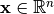, which consists of a conditional embedding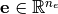and an unconditional input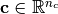, i.e.,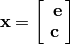We simply define the variance of an inputas the weighted average of the individual variances, i.e.,To see that this is correct, consider a linear layer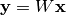or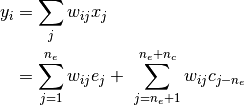Hence, we can compute the variance of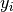as follows (assuming the typical Xavier assumptions):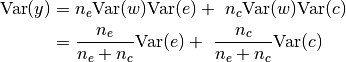Note, that Xavier would have initializedusing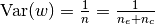.

Parameters:
• method (str) –

The type of initialization that should be applied. Possible options are:

• 'in': Use Hyperfan-in.

• 'out': Use Hyperfan-out.

• 'harmonic': Use the harmonic mean of the Hyperfan-in and Hyperfan-out init.

• use_xavier (bool) – Whether Kaiming (False) or Xavier (True) init should be used.

• uncond_var (float) – The variance of unconditional embeddings. This value is only taken into consideration if uncond_in_size > 0 (cf. constructor arguments).

• cond_var (float) – The initial variance of conditional embeddings. This value is only taken into consideration if cond_in_size > 0 (cf. constructor arguments).

• mnet (mnets.mnet_interface.MainNetInterface, optional) – If applicable, the user should provide the main (or target) network, whose weights are generated by this hypernetwork. The mnet instance is used to extract valuable information that improve the initialization result. If provided, it is assumed that target_shapes (cf. constructor arguments) corresponds either to mnets.mnet_interface.MainNetInterface.param_shapes or mnets.mnet_interface.MainNetInterface.hyper_shapes_learned.

• w_val (list or dict, optional) –

The mean of the distribution with which output head weight matrices are initialized. Note, each weight tensor prescribed by hnets.hnet_interface.HyperNetInterface.target_shapes is produced via an independent linear output head.

One may either specify a list of numbers having the same length as hnets.hnet_interface.HyperNetInterface.target_shapes or specify a dictionary which may have as keys the tensor names occurring in mnets.mnet_interface.MainNetInterface.param_shapes_meta and the corresponding mean value for the weight matrices of all output heads producing this type of tensor. If a list is provided, entries may be None and if a dictionary is provided, not all types of parameter tensors need to be specified. For tensors, for which no value is specified, the default value will be used. The default values for tensor types 'weight' and 'bias' are calculated based on the proposed hyperfan-initialization. For other tensor types the actual hypernet outputs should be drawn from the following distributions

• 'bn_scale':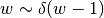• 'bn_shift':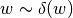• 'cm_scale':• 'cm_shift':• 'embedding':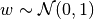Which would correspond to the following passed arguments

w_val = {
'bn_scale': 0,
'bn_shift': 0,
'cm_scale': 0,
'cm_shift': 0,
'embedding': 0
}
w_var = {
'bn_scale': 0,
'bn_shift': 0,
'cm_scale': 0,
'cm_shift': 0,
'embedding': 0
}
b_val = {
'bn_scale': 1,
'bn_shift': 0,
'cm_scale': 1,
'cm_shift': 0,
'embedding': 0
}
b_var = {
'bn_scale': 0,
'bn_shift': 0,
'cm_scale': 0,
'cm_shift': 0,
'embedding': 1
}


• w_var (list or dict, optional) – The variance of the distribution with which output head weight matrices are initialized. Variance values of zero means that weights are set to a constant defined by w_val. See description of argument w_val for more details.

• b_val (list or dict, optional) – The mean of the distribution with which output head bias vectors are initialized. See description of argument w_val for more details.

• b_var (list or dict, optional) – The variance of the distribution with which output head bias vectors are initialized. See description of argument w_val for more details.

distillation_targets()[source]

Targets to be distilled after training.

See docstring of abstract super method mnets.mnet_interface.MainNetInterface.distillation_targets().

This network does not have any distillation targets.

Returns:

None

forward(uncond_input=None, cond_input=None, cond_id=None, weights=None, distilled_params=None, condition=None, ret_format='squeezed')[source]

Compute the weights of a target network.

Parameters:
• (....) – See docstring of method hnets.hnet_interface.HyperNetInterface.forward().

• condition (int, optional) – This argument will be passed as argument stats_id to the method utils.batchnorm_layer.BatchNormLayer.forward() if batch normalization is used.

Returns:

See docstring of method hnets.hnet_interface.HyperNetInterface.forward().

Return type:

(list or torch.Tensor)

get_cond_in_emb(cond_id)[source]

Get the cond_id-th (conditional) input embedding.

Parameters:

cond_id (int) – Determines which input embedding should be returned (the ID has to be between 0 and num_cond_embs-1, where num_cond_embs denotes the corresponding constructor argument).

Returns:

(torch.nn.Parameter)

training: bool

## Example Instantiations of a Structured Chunked MLP - Hypernetwork

The module hnets.structured_hmlp_examples provides helpers for example instantiations of hnets.structured_mlp_hnet.StructuredHMLP.

Functions in this module typically take a given main network and produce the constructor arguments chunk_shapes, num_per_chunk and assembly_fct of class hnets.structured_mlp_hnet.StructuredHMLP.

Note

These examples should be used with care. They are meant as inspiration and might not cover all possible usecases.

 Design a structured chunking for a ResNet. Design a structured chunking for a Wide-ResNet (WRN).
hypnettorch.hnets.structured_hmlp_examples.resnet_chunking(net, gcd_chunking=False)[source]

Design a structured chunking for a ResNet.

A resnet as implemented in class mnets.resnet.ResNet consists roughly of 5 parts:

• An input convolutional layer with weight shape [C_1, C_in, 3, 3]

• 3 blocks of 2*n convolutional layers each where the first layer has shape [C_i, C_j, 3, 3] with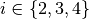and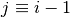and the remaining 2*n-1 layers have a weight shape of [C_i, C_i, 3, 3].

• A final fully connected layer of shape [n_classes, n_hidden].

Each layer may additionally have a bias vector and (if batch normalization is used) a scale and shift vector.

For instance, if a resnet with biases and batchnorm is used and the first layer will be produced as one structured chunk, then the first chunk shape (see return value chunk_shapes) will be: [[C_1, C_in, 3, 3], [C_1], [C_1], [C_1]].

This function will chunk layer wise (i.e., a chunk always comprises up to 4 elements: weights tensor, bias vector, batchnorm scale and shift). By default, layers with the same shape are grouped together. Hence, the standard return value contains 8 chunk shapes (input layer, first layer of each block, remaining layers of each block (which all have the same shape) and the fully-connected output layer). Therefore, the return value num_per_chunk would be as follows: [1, 1, 2*n-1, 1, 2*n-1, 1, 2*n-1, 1].

Parameters:
• net (mnets.resnet.ResNet) – The network for which the structured chunking should be devised.

• gcd_chunking (bool) –

If True, the layers within the 3 resnet blocks will be produced by 4 chunks. Therefore, the greatest common divisor (gcd) of the feature sizes C_1, C_2, C_3, C_4 is computed and the 6 middle chunk_shapes produced by default are replaced by 4 chunk shapes [[C_gcd, C_i, 3, 3], [C_gcd]] (assuming no batchnorm is used). Note, the first and last entry of chunk_shapes will remain unchanged by this option.

Hence, len(num_per_chunk) = 6 in this case.

Returns:

Tuple containing the following arguments that can be passed to the constructor of class hnets.structured_mlp_hnet.StructuredHMLP.

• chunk_shapes (list)

• num_per_chunk (list)

• assembly_fct (func)

Return type:

(tuple)

hypnettorch.hnets.structured_hmlp_examples.wrn_chunking(net, ignore_bn_weights=True, ignore_out_weights=True, gcd_chunking=False)[source]

Design a structured chunking for a Wide-ResNet (WRN).

This function is in principle similar to function resnet_chunking(), but with the goal to provide a chunking scheme that is identical to the one proposed in (accessed August 18th, 2020):

Sacramento et al., “Economical ensembles with hypernetworks”, 2020 https://arxiv.org/abs/2007.12927

Therefore, a WRN as implemented in class mnets.wide_resnet.WRN is required. For instance, a WRN-28-10-B(3,3) can be instantiated as follows, using batchnorm but no biases in all convolutional layers:

wrn = WRN(in_shape=(32, 32, 3), num_classes=10, n=4, k=10,
num_feature_maps=(16, 16, 32, 64), use_bias=False,
use_fc_bias=True, no_weights=False, use_batch_norm=True)


We denote channel sizes by [C_in, C_1, C_2, C_3, C_4], where C_in is the number of input channels and the remaining C_1, C_2, C_3, C_4 denote the channel size per convolutional group. The widening factor is denoted by k.

In general, there will be up to 11 layer groups, which will be realized by separate hypernetworks (cmp table S1 in Sacramento et al.):

• 0: Input layer weights. If the network’s convolutional layers have biases and batchnorm layers while ignore_bn_weights=False, then this hypernet will produce weights of shape [[C_1, C_in, 3, 3], [C_1], [C_1], [C_1]]. However, without convolutional bias terms and with ignore_bn_weights=True, the hypernet will only produce weights of shape [[C_1, C_in, 3, 3]]. This specification applies to all layer groups generating convolutional layers.

• 1: This layer group will generate the weights of the first convolutional layer in the first convolutional group, e.g., [[k*C_2, C_1, 3, 3]]. Let’s define r = max(k*C_2/C_1, C_1/k*C_2). If r=1 or r=2 or gcd_chunking=True, then this group is merged with layer group 2.

• 2: The remaining convolutional layer of the first convolutional group. If r=1, r=2 or gcd_chunking=True, then all convolutional layers of the first group are generated. However, if biases or batch norm weights have to be generated, then this form of chunking leads to redundancy. Imagine bias terms are used and that the first layer in this convolutional group has weights [[160, 16, 3, 3], ], while the remaining layers have shape [[160, 160, 3, 3], ]. If that’s the case, the hypernetwork output will be of shape [[160, 16, 3, 3], ], meaning that 10 chunks have to be produced for each except the first layer. However, this means that per convolutional layer 10 bias vectors are generated, while only one is needed and therefore the other 9 will go to waste.

• 3: Same as 1 for the first layer in the second convolutional group.

• 4 (labelled as 3 in the paper): Same as 2 for all convolutional layers (potentially excluding the first) in the second convolutional group.

• 5: Same as 1 for the first layer in the third convolutional group.

• 6 (labelled as 4 in the paper): Same as 2 for all convolutional layers (potentially excluding the first) in the third convolutional group.

• 7 (labelled as 5 in the paper): If existing, this hypernetwork produces the 1x1 convolutional layer realizing the residual connection connecting the first and second residual block in the first convolutional group.

• 8 (labelled as 6 in the paper): Same as 7 but for the first residual connection in the second convolutional group.

• 9 (labelled as 7 in the paper): Same as 7 but for the first residual connection in the third convolutional group.

• 10: This hypernetwork will produce the weights of the fully connected output layer, if ignore_out_weights=False.

Thus, the WRN weights would maximally be produced by 11 different sub- hypernetworks.

Note

There is currently an implementation mismatch, such that the implementation provided here does not 100% mimic the architecture described in Sacramento et al..

To be specific, given the wrn generated above, the hypernetwork output for layer group 2 will be of shape [160, 160, 3, 3], while the paper expects a vertical chunking with a hypernet output of shape [160, 80, 3, 3].

Parameters:
• net (mnets.wide_resnet.WRN) – The network for which the structured chunking should be devised.

• ignore_bn_weights (bool) – If True, even if the given net has batchnorm weights, they will be ignored by this function.

• ignore_out_weights (bool) – If True, output weights (layer group 10) will be ignored by this function.

• gcd_chunking (bool) – If True, layer groups 1, 3 and 5 are ignored. Instead, the greatest common divisor (gcd) of input and output feature size in a convolutional group is computed and weight tensors within a convolutional group (i.e., layer groups 2, 4 and 6) are chunked according to this value. However, note that this will cause the generation of unused bias and batchnorm weights if existing (cp. description of layer group 2).

Returns:

Tuple containing the following arguments that can be passed to the constructor of class hnets.structured_mlp_hnet.StructuredHMLP.

• chunk_shapes (list)

• num_per_chunk (list)

• assembly_fct (func)

Return type:

(tuple)

## Structured Chunked MLP - Hypernetwork

The module hnets.structured_mlp_hnet contains a Structured Chunked Hypernetwork, i.e., a hypernetwork that is aware of the target network architecture and choses a smart way of chunking.

In contrast to the Chunked Hypernetwork hnets.chunked_mlp_hnet.ChunkedHMLP, which just flattens the target_shapes and splits them into equally sized chunks (ignoring the underlying network structure in terms of layers or type of weight (bias, kernel, …)), the StructuredHMLP aims to preserve this structure when chunking the target weights.

Example

Assume target_shapes = [, , [10, 5], , [20, 5], ].

There are now many ways to split those weights into chunks. In the simplest case, we consider only one chunk and produce all weights at once with a Full Hypernetwork hnets.mlp_hnet.HMLP.

Another simple scenario would be to realize that all shapes except the first two are different. So, we create a total of 5 internal hypernetworks for those 6 weight tensors, where the first internal hypernetwork would produce weights of shape  upon receiving an external input plus an internal chunk embedding. See below for an example instantiation:

def assembly_fct(list_of_chunks):
assert len(list_of_chunks) == 4
ret = []
for chunk in list_of_chunks:
ret.extend(chunk)
return ret

hnet = StructuredHMLP([, , [10, 5], , [20, 5], ],
[[], [[10, 5], ], [[20, 5], ]], [2, 1, 1], 8,
{'layers': [10,10]}, assembly_fct, cond_chunk_embs=True,
uncond_in_size=0, cond_in_size=0, verbose=True,
no_uncond_weights=False, no_cond_weights=False, num_cond_embs=1)


A smarter way of chunking would be to realize that the last two shapes are just twice the middle two shapes. Hence, we could instantiate two internal hypernetworks. The first one would be used to produce tensors of shape  and therefore require 2 chunk embeddings. The second internal hypernetwork would be used to create tensors of shape [10, 5], , requiring 3 chunk embeddings (the last two chunks together make up the last two target tensors of shape [20, 5], ).

def assembly_fct(list_of_chunks):
assert len(list_of_chunks) == 5
ret = [*list_of_chunks, *list_of_chunks, *list_of_chunks]
for t, tensor in enumerate(list_of_chunks):
ret.append(torch.cat([tensor, list_of_chunks[t]], dim=0))
return ret

hnet = StructuredHMLP([, , [10, 5], , [20, 5], ],
[[], [[10, 5], ]], [2, 3], 8,
{'layers': [10,10]}, assembly_fct, cond_chunk_embs=True,
uncond_in_size=0, cond_in_size=0, verbose=True,
no_uncond_weights=False, no_cond_weights=False, num_cond_embs=1)


Example

This hypernetwork can also be used to realize soft-sharing via templates as proposed in Savarese et al.

Assume a target network with 3 layers of identical weight shapes target_shapes=[s, s, s], where s denotes a weight shape.

If we want to create these 3 weight tensors via a linear combination of two templates, we could create an instance of StructuredHMLP as follows:

def assembly_fct(list_of_chunks):
assert len(list_of_chunks) == 3
return [list_of_chunks, list_of_chunks,
list_of_chunks]

hnet = StructuredHMLP([s, s, s], [[s]], , 2,
{'layers': [], 'use_bias': False}, assembly_fct
cond_chunk_embs=True, uncond_in_size=0, cond_in_size=0,
verbose=True, no_uncond_weights=False, no_cond_weights=False,
num_cond_embs=1)


There will be one underlying linear hypernetwork, that expects a 2-dimensional embedding input. The computation of the linear hypernetwork can be seen as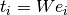. Where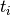is a tensor of shape s containing the weights of the-th chunk (with chunk embedding).

The 2 templates are encoded in the hypernetwork weights, whereas the chunk embedding represents the coefficients of the linear combination.

class hypnettorch.hnets.structured_mlp_hnet.StructuredHMLP(target_shapes, chunk_shapes, num_per_chunk, chunk_emb_sizes, hmlp_kwargs, assembly_fct, cond_chunk_embs=False, uncond_in_size=0, cond_in_size=8, verbose=True, no_uncond_weights=False, no_cond_weights=False, num_cond_embs=1)[source]

Implementation of a structured chunked fully-connected hypernet.

This network builds a series of full hypernetworks internally (hidden from the user). There will be one internal hypernetwork for each element of chunk_shapes. Those internal hypernetworks can produce an arbitrary amount of chunks (as defined by num_per_chunk). All those chunks are finally assembled by function assembly_fct to produce tensors according to target_shapes.

Note

It is possible to set uncond_in_size and cond_in_size to zero if cond_chunk_embs is True and there are no zeroes in argument chunk_emb_sizes.

Parameters:
• (....) – See constructor arguments of class hnets.mlp_hnet.HMLP.

• chunk_shapes (list) – List of lists of lists of integers. Each chunk will be produced by its own internal hypernetwork (instance of class hnets.mlp_hnet.HMLP). Hence, this list can be seen as a list of target_shapes, passed to the underlying internal hypernets.

• num_per_chunk (list) – List of the same length as chunk_shapes, that determines how often each of these chunks has to be produced.

• chunk_emb_sizes (list or int) –

List with the same length as chunk_shapes or single integer that will be expanded to this length. Determines the chunk embedding size per internal hypernetwork.

Note

Embeddings will be initialized with a normal distribution using zero mean and unit variance.

Note

If the corresponding entry in num_per_chunk is 1, then an embedding size might be 0, which means there won’t be chunk embeddings for the corresponding internal hypernetwork.

• hmlp_kwargs (list or dict) –

List of dictionaries or a single dictionary that will be expanded to such a list. Those dictionaries may contain keyword arguments for each instance of class hnets.mlp_hnet.HMLP that will be generated.

The following keys are not permitted in these dictionaries: - uncond_in_size - cond_in_size - no_uncond_weights - no_cond_weights - num_cond_embs Those arguments will be determined by the corresponding keyword arguments of this class!

• assembly_fct (func) –

A function handle that takes the produced chunks and converts them into tensors with shapes target_shapes.

The function handle must have the signature: assembly_fct(list_of_chunks). The argument list_of_chunks is a list of lists of tensors. The function is expected to return a list of tensors, each of them having a shape as specified by target_shapes.

Example

Assume chunk_shapes=[[], [[10, 5], ]] and num_per_chunk=[2, 1]. Then the argument list_of_chunks will be a list of lists of tensors as follows: [[tensor(3)], [tensor(3)], [tensor(10, 5), tensor(5)]].

If target_shapes=[, , [10, 5], ], then the output of assembly_fct is expected to be a list of tensors as follows: [tensor(3), tensor(3), tensor(10, 5), tensor(5)].

Note

This function considers one sample at a time, even if a batch of inputs is processed.

Note

It is assumed that assembly_fct does not further process the incoming weights. Otherwise, the attributes mnets.mnet_interface.MainNetInterface.has_fc_out and mnets.mnet_interface.MainNetInterface.has_linear_out might be invalid.

• cond_chunk_embs (bool) – See documentation of class hnets.chunked_mlp_hnet.ChunkedHMLP

Initializes internal Module state, shared by both nn.Module and ScriptModule.

property chunk_emb_shapes

List of lists of integers. The list contains the shape of the chunk embeddings required per forward sweep.

Note

Some internal hypernets might not need chunk embeddings if the corresponding entry in chunk_emb_sizes is zero.

Type:

list

property cond_chunk_embs

Whether chunk embeddings are unconditional (False) or conditional (True) parameters. See constructor argument cond_chunk_embs.

Type:

bool

distillation_targets()[source]

Targets to be distilled after training.

See docstring of abstract super method mnets.mnet_interface.MainNetInterface.distillation_targets().

This network does not have any distillation targets.

Returns:

None

forward(uncond_input=None, cond_input=None, cond_id=None, weights=None, distilled_params=None, condition=None, ret_format='squeezed')[source]

Compute the weights of a target network.

Parameters:
• (....) – See docstring of method hnets.mlp_hnet.HMLP.forward().

• weights (list or dict, optional) –

If provided as dict and chunk embeddings are considered conditional (see constructor argument cond_chunk_embs), then the additional key chunk_embs can be used to pass a batch of chunk embeddings. This option is mutually exclusive with the option of passing cond_id. Note, if conditional inputs via cond_input are expected, then the batch sizes must agree.

A batch of chunk embeddings is expected to be a list of tensors of shape [B, *ce_shape], where B denotes the batch size and ce_shape is a shape from list chunk_emb_shapes.

Returns:

See docstring of method hnets.hnet_interface.HyperNetInterface.forward().

Return type:

(list or torch.Tensor)

get_chunk_embs(cond_id=None)[source]

Get the chunk embeddings.

Parameters:

cond_id (int) – Is mandatory if constructor argument cond_chunk_embs was set. Determines the set of chunk embeddings to be considered.

Returns:

A list of tensors with shapes prescribed by chunk_emb_shapes.

Return type:

(list)

get_cond_in_emb(cond_id)[source]

Get the cond_id-th (conditional) input embedding.

Parameters:

(....) – See docstring of method hnets.mlp_hnet.HMLP.get_cond_in_emb().

Returns:

(torch.nn.Parameter)

property internal_hnets

The list of internal hypernetworks (instances of class hnets.mlp_hnet.HMLP) which are created to produce the individual chunks according to constructor argument chunk_shapes.

Type:

list

property num_chunks

The total number of chunks that make up the hypernet output.

This attribute simply corresponds to np.sum(num_per_chunk)`.

Type:

int

training: bool